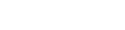• Home
• DU JAT Inverse Trigo...

# DU JAT Inverse Trigonometric Functions Questions

Author : Palak Khanna

August 16, 2022

SHARE

Inverse trigonometric functions are known as the inverse functions of basic trigonometric functions that are sine, cosine, tangent, cotangent, cosecant, and secant functions. These functions are majorly used in the field of engineering, physics, geometry and navigation.

In most of the entrance examinations after 12t standard, the Inverse Trigonometric functions topic is included in the Mathematics section.

## What are Inverse Trigonometric Functions?

The inverse trigonometric functions perform the opposite function of trigonometric functions that are sine, cosine, tangent, cotangent, cosecant, and secant. They are also known as "Arc Functions" as for a given value of trigonometric functions they provide the length of the arc needed to obtain that particular value.

If you are worried about what type of Inverse Trigonometric Function Questions are asked in DU JAT, then this post shall take you through most commonly asked Inverse Trigonometric Function Questions along with solutions.

### Basic Inverse Trigonometric Function Formulas

 Inverse Trig Functions Formulas Arcsine sin-1(-x) = -sin-1(x), x ∈ [-1, 1] Arccosine cos-1(-x) = π -cos-1(x), x ∈ [-1, 1] Arctangent tan-1(-x) = -tan-1(x), x ∈ R Arccotangent cot-1(-x) = π – cot-1(x), x ∈ R Arcsecant sec-1(-x) = π -sec-1(x), |x| ≥ 1 Arccosecant cosec-1(-x) = -cosec-1(x), |x| ≥ 1

### Inverse Trigonometric Function Questions and Answers for DU JAT

Here is the list of sample Inverse Trigonometric Function questions curated from previous year DU JAT Question Papers. Our expert from Supergrads has solved the questions. Go through the questions along with detailed solutions and enhance your preparation for the upcoming DU JAT exam.

1. Find the value of cos-1(1/2) + 2 sin-1(1/2)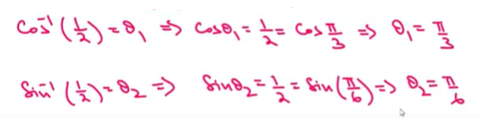π/3 + π/6 = 2π/3

2. Find the value of tan-1(2 cos (2 sin-1(-1/2)))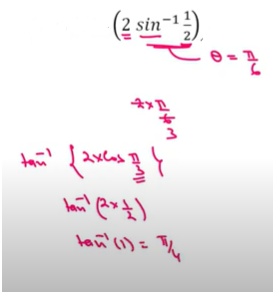3. Find the value of tan-1(√3) + sec-1(-2)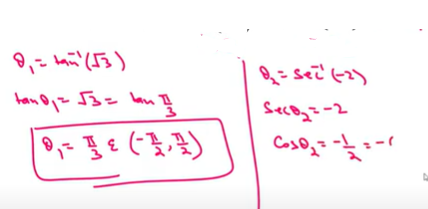4. Find the value of Sin [π/3 - sin-1(-1/2)]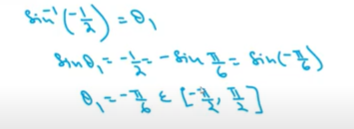Sin [π/3+π/6] = Sin (π/2) = 15. Find the value of Sin (1/2 cos-1 4/5)

Let us consider: Sin (1/2 cos-1 4/5) = SinΘ/2 ; cos-1 4/5 = cosΘ = 4/5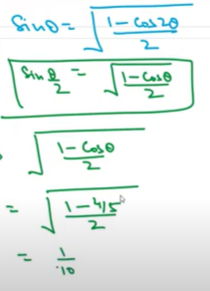6. Evaluate Sin(2 cos-1 (-3/5) )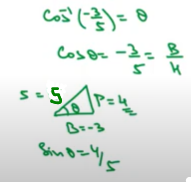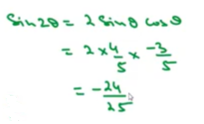### Inverse Trigonometric Functions Questions PDF

For more questions on Inverse Trigonometric questions, download the pdf below which includes questions and solutions. Enhance your preparation for DU JAT by solving these questions and score good marks in the mathematics section.

• Home
• DU JAT Inverse Trigo...

# DU JAT Inverse Trigonometric Functions Questions

Author : Palak Khanna

Updated On : August 16, 2022

SHARE

Inverse trigonometric functions are known as the inverse functions of basic trigonometric functions that are sine, cosine, tangent, cotangent, cosecant, and secant functions. These functions are majorly used in the field of engineering, physics, geometry and navigation.

In most of the entrance examinations after 12t standard, the Inverse Trigonometric functions topic is included in the Mathematics section.

## What are Inverse Trigonometric Functions?

The inverse trigonometric functions perform the opposite function of trigonometric functions that are sine, cosine, tangent, cotangent, cosecant, and secant. They are also known as "Arc Functions" as for a given value of trigonometric functions they provide the length of the arc needed to obtain that particular value.

If you are worried about what type of Inverse Trigonometric Function Questions are asked in DU JAT, then this post shall take you through most commonly asked Inverse Trigonometric Function Questions along with solutions.

### Basic Inverse Trigonometric Function Formulas

 Inverse Trig Functions Formulas Arcsine sin-1(-x) = -sin-1(x), x ∈ [-1, 1] Arccosine cos-1(-x) = π -cos-1(x), x ∈ [-1, 1] Arctangent tan-1(-x) = -tan-1(x), x ∈ R Arccotangent cot-1(-x) = π – cot-1(x), x ∈ R Arcsecant sec-1(-x) = π -sec-1(x), |x| ≥ 1 Arccosecant cosec-1(-x) = -cosec-1(x), |x| ≥ 1

### Inverse Trigonometric Function Questions and Answers for DU JAT

Here is the list of sample Inverse Trigonometric Function questions curated from previous year DU JAT Question Papers. Our expert from Supergrads has solved the questions. Go through the questions along with detailed solutions and enhance your preparation for the upcoming DU JAT exam.

1. Find the value of cos-1(1/2) + 2 sin-1(1/2)π/3 + π/6 = 2π/3

2. Find the value of tan-1(2 cos (2 sin-1(-1/2)))3. Find the value of tan-1(√3) + sec-1(-2)4. Find the value of Sin [π/3 - sin-1(-1/2)]Sin [π/3+π/6] = Sin (π/2) = 15. Find the value of Sin (1/2 cos-1 4/5)

Let us consider: Sin (1/2 cos-1 4/5) = SinΘ/2 ; cos-1 4/5 = cosΘ = 4/56. Evaluate Sin(2 cos-1 (-3/5) )### Inverse Trigonometric Functions Questions PDF

For more questions on Inverse Trigonometric questions, download the pdf below which includes questions and solutions. Enhance your preparation for DU JAT by solving these questions and score good marks in the mathematics section.